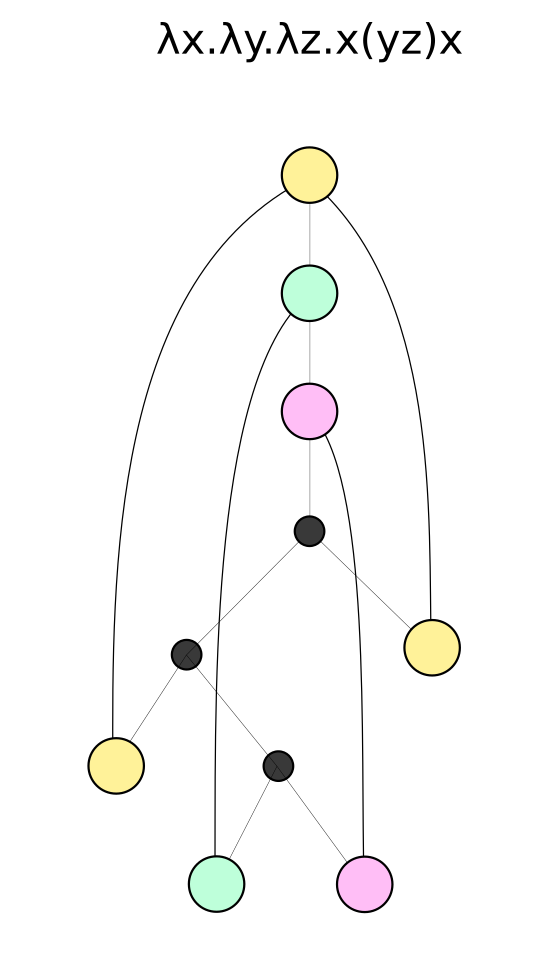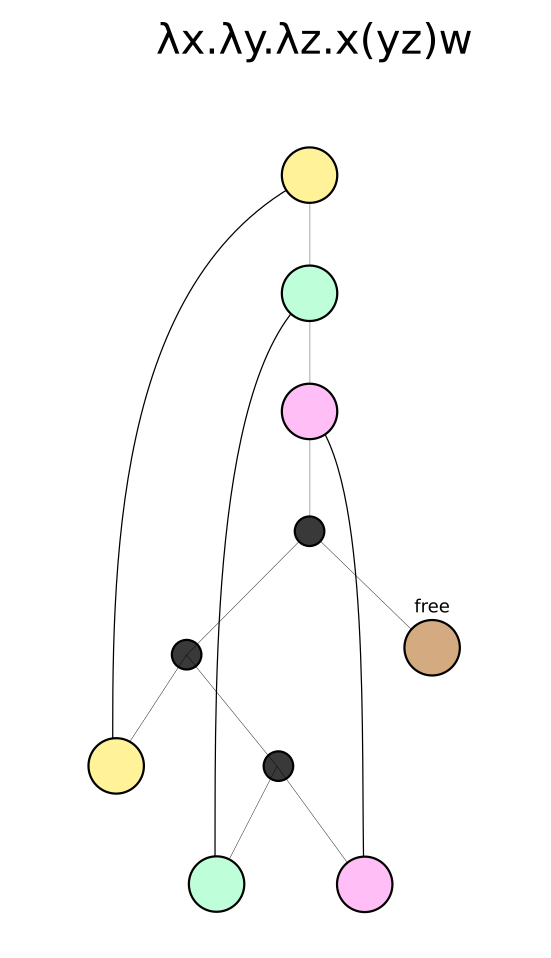Show Question
Math and science::Theory of Computation::Lambda calculus

# Lamba terms. Definition.

λ-terms are the elementary objects of λ-calculus. Below, they are defined first in the traditional way (as sequences) and then as trees.

### λ-terms. Defined as sequences.

First, assume the existence of an infinite sequence of term-variables. Term-variables are denoted using "u", "v", "w", "x", "y", "z", with optional subscripts. The set of λ-terms is then inductively defined as follows:

1. each term-variable is a λ-term, called an atomic term;
2. if $$M$$ and $$N$$ are λ-terms, then $$(MN)$$ is a λ-term, called an application;
3. if $$x$$ is a term-variable and $$M$$ is a λ-term, then $$(\lambda x.\ M )$$ is a λ-term, called a λ-abstract.

A composite λ-term is a λ-term that is not an atomic term.

This definition raises some questions. See the reverse for more details, including an alternative.

The above definition treats λ-terms as sequences (one element following another) in a 1D sense, like a sequence of integers. However, a more natural model for λ-terms is that of a tree. The next definition tries to capture this idea.

### λ-terms. Defined as trees.

A lambda term is a tree where:

• Nodes may have 0, 1 or 2 children.
• Every 0-child node has a mapping to an ancestor 1-child node.

0-child nodes are called leaf nodes, 1-child nodes are called abstraction nodes, and 2-child nodes are called application nodes.

The reverse side has visualization for the trees. Can you remember them?

This definition is not exactly formal. For example, what is a tree? What are nodes? However, at least these deficiency's are not hidden, like how the reliance on the meaning of character sequences from an alphabet of variable-symbols seems to be hidden in the first definition.

#### Free nodes?

This definition doesn't allow non-closed terms. The reverse side has an alternative definition that tries to address this second point. Can you remember how it's done?

### Remarks on the traditional definition

This definition raises some questions.

• can we just assert the existence of an infinite sequence of "variables"? This is like saying "let there be a function from the natural numbers to the set of "variables". Which avoids actually defining the set of variables, and implicitly assumes the definition of a lot of math, such as natural numbers, sequences and cardinality (what else?). It makes the sequence of variables countable, yet the set of variables either finite, countable or uncountable; however, it seems like countable is what is trying to be implied here.
• The second point blurs the line between objects an notation. Are $$M$$ and $$N$$ labels for set elements, and is $$(MN)$$ another label for a set element? What is equality between set elements? No, this is too troublesome. I think $$M$$ is meta-notation (what's the right term?) to act as a placeholder in a sequence, and $$(MN)$$ is literally a sequence "(", "M", "N", ")", where M and N are in some sense allowed to vary over the set of variables.

#### Alternative sequence definition

Below is an alternative definition, which I feel has stronger footing. It exists in context of alphabets, languages, grammars etc.

### λ-terms. Defined with grammars.

Let $$V$$ be a (in)finite (can it be countably infinite?) alphabet. Then the set of λ-terms are those defined by the grammar:

$\Lambda = V|(\Lambda \Lambda)|(\lambda V .\ \Lambda )$

### Alternative tree definition

Below is an alternative definition using trees. It tries to allow for free nodes.

### λ-terms. Defined as trees with natural number functions.

A lambda term is a tree where:

• Nodes may have 0, 1 or 2 children.
• Every 0-child node has a mapping to a positive integer.

Leaf, abstraction and application nodes are defined as before.

The natural number mapping (instead of the mapping to parent abstraction nodes) encodes a map to a parent abstraction node by counting up through ancestor nodes. In addition, we are able to set counts that go beyond the root of the tree; this is how we can create the sense of the unbound nodes.

The positive integer represents the count up the 1-child ancestor list. The count can go past the root node, and in this way, it will represent unbound nodes. They are not completely "unbound", in that, while they don't know what node they are bound to, they do know what level they are bound to.

### Tree visualizations

An tree representation:A representation with a free variable: